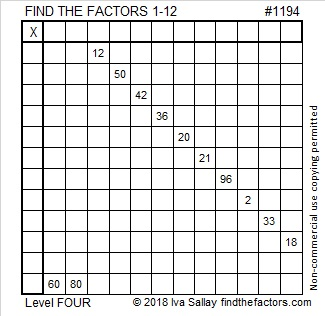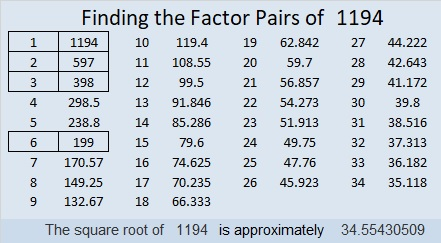# 1194 and Level 4

The more multiplication facts you know, the easier these puzzles become. Working on these puzzles can help you learn the multiplication table better. Go ahead,  give this puzzle a try!Print the puzzles or type the solution in this excel file: 12 factors 1187-1198

Here are a few facts about the number 1194:

• 1194 is a composite number.
• Prime factorization: 1194 = 2 × 3 × 199
• The exponents in the prime factorization are 1, 1, and 1. Adding one to each and multiplying we get (1 + 1)(1 + 1)(1 + 1) = 2 × 2 × 2 = 8. Therefore 1194 has exactly 8 factors.
• Factors of 1194: 1, 2, 3, 6, 199, 398, 597, 1194
• Factor pairs: 1194 = 1 × 1194, 2 × 597, 3 × 398, or 6 × 199
• 1194 has no square factors that allow its square root to be simplified. √1194 ≈ 34.55431194 is the sum of consecutive prime numbers two ways:
131 + 137 + 139 + 149 + 151 + 157 + 163 + 167 = 1194
283 + 293 + 307 + 311 = 1194

1194 is palindrome 424 in BASE 17

This site uses Akismet to reduce spam. Learn how your comment data is processed.